C++11 新特性详解 1一览表

42 篇文章 2 订阅

1.explicit关键字

2.左值和右值概念

3.函数返回当引用

4.C++11 _array容器用法

5.C++类型转换简介

5.1.static_cas转换

5.2.reinterpreter_cast 转换

5.3.dynamic_cast转换

5.4.const_cast 转换

1.explicit关键字

explicit   /ɪkˈsplɪsɪt/   明确的；清楚的；直率的；详述的

class student {
public:
student(int age) {
this->age = age;
cout << "age=" << age << endl;
}
student(int age, string name) {
this->age = age;
this->name = name;
cout << "age=" << age << "  name=" << name << endl;
}
~student() {

}
int getAge() {
return this->age;
}
string getName() {
return this->name;
}
private:
string name;
int age;
};

int main() {
//显示构造，直接在括号里面写
student xiaoM(18);

//显示构造
student xiaoH(19, "小花");

//隐式构造
student xiaoW = 18;

//隐式构造（C++11前编译器无法通过）
student xiaoZ = { 19,"小张" };

system("pause");
return 0;
}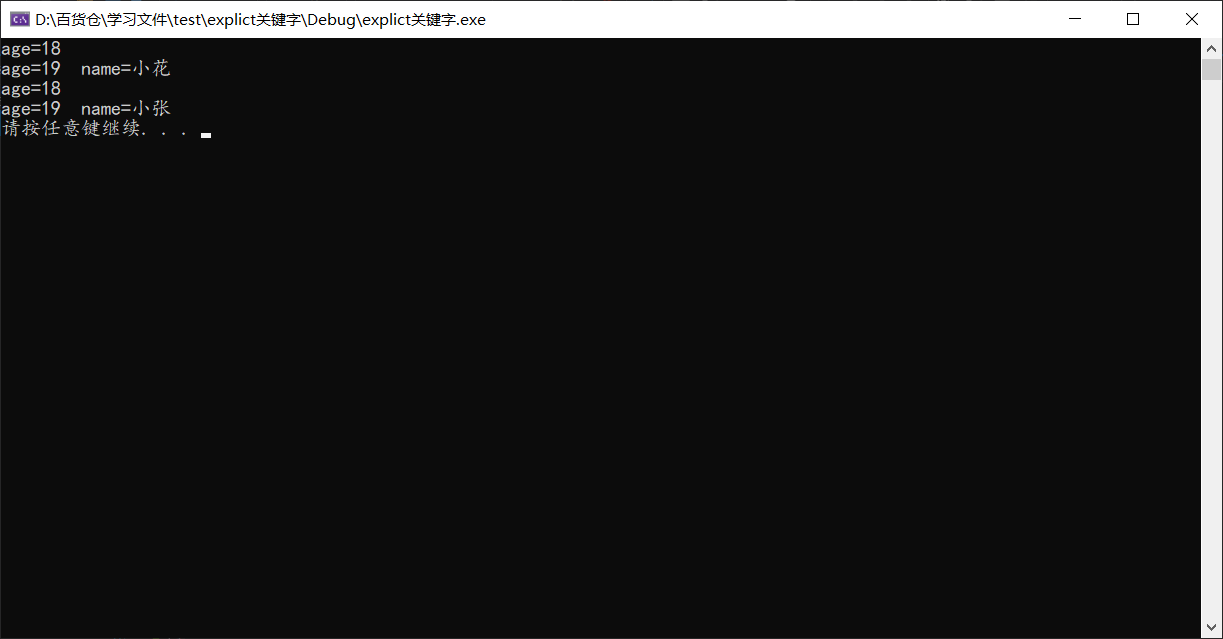class student {
public:
explicit student(int age) {
this->age = age;
cout << "age=" << age << endl;
}
private:
string name;
int age;
};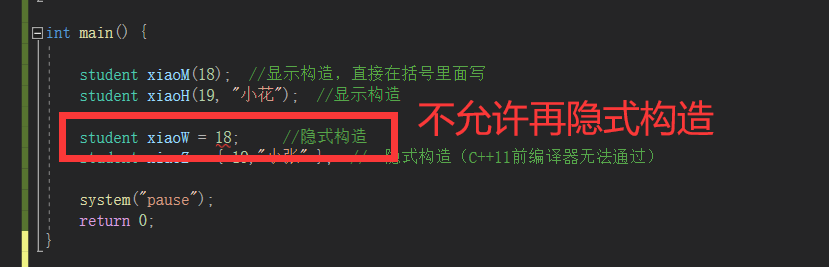explicit关键字的作用在于：在实际开发中 等号会产生一些歧义，等号本身又有赋值的作用，无法在一瞬间辨别等号是用于赋值还是调用隐式构造，不便于阅读。

2.左值和右值概念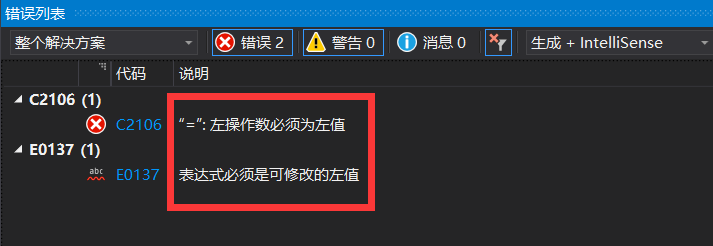#include<iostream>
using namespace std;

int demo() {
int i = 0;
return i;
}

int main() {

//将函数返回作为左值
demo() = 888;
return 0;
}

存储层次结构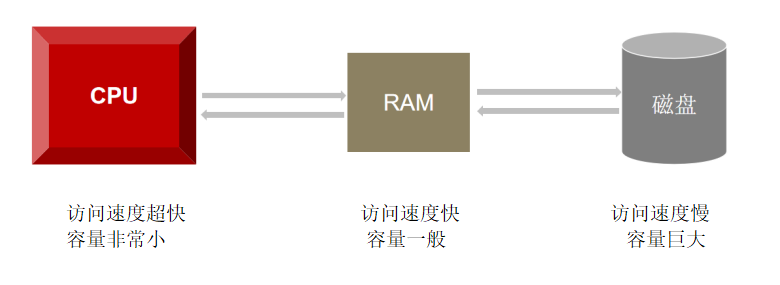a 和 b 的值会保存在内存中，当程序遇到c = a + b，会将a和b的值拿到cpu的寄存器中，再将计算结果返回给内存中的c，当我们Ctrl + S后，内存中的数据就可以永久保存到磁盘中。

左值和右值的概念

按字面意思，通俗地说。以赋值符号 = 为界，等号（=）左边的就是左值（lvalue），等号（=） 右边就是右值(rvalue)。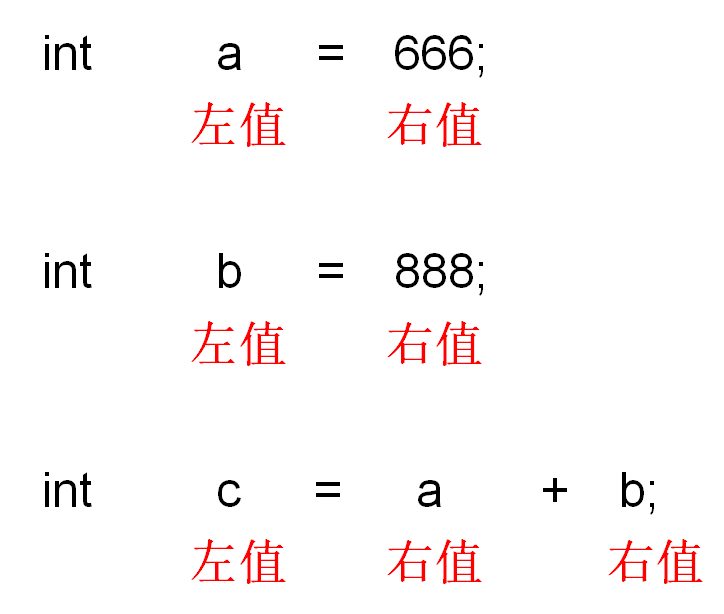lvalue —— 代表一个在内存中占有确定位置的对象（换句话说就是有一个地址）。
rvalue —— 通过排他性来定义，每个表达式不是lvalue就是rvalue。因此从上面的   lvalue的定义，rvalue是在不在内存中占有确定位置的表达式，而是存在在寄存器中。

我们将下面程序转为汇编码观察

#include<iostream>
using namespace std;

int main() {
int a = 1;
int b = 2;
int c = 3;

c = a + b;
printf("%d", c);
return 0;
}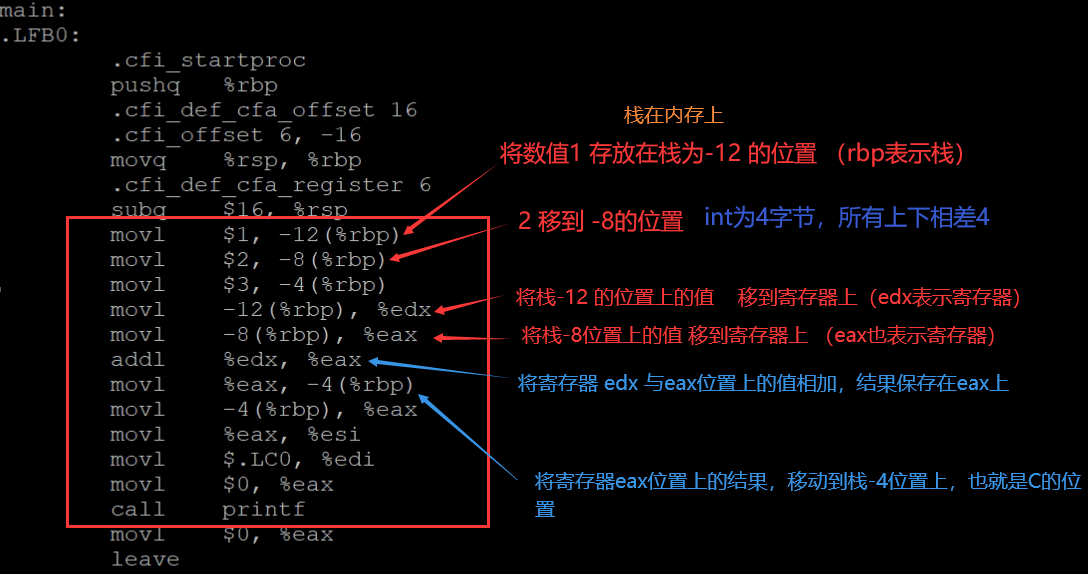a + b的结果保存在寄存器中，再将寄存器的值返回给内存

3.函数返回当引用

上面我们讲了左值与右值，当我们把函数返回值作为左值时，编译器给出报错提示，而C++11的新增特性“函数返回引用”又可以实现函数返回值作为左值的操作

C++引用使用时的难点：

1.当函数返回参数是引用时

若返回栈变量（自动变量/临时变量），不能成为其它引用的初始值，不能作为左值使用。

2. 若返回静态变量或全局变量

·可以成为其他引用的初始值

·即可作为右值使用，也可作为左值使用

3.   返回形参当引用

(注：C++链式编程中，经常用到引用，运算符重载专题） 例如在重载“<<"左移运算符后，我们想要链式输出，就是返回的cout引用

1. 函数返回值为引用，返回临时变量作为其他引用的初始值

#include<iostream>
using namespace std;

int& demo() {
int i = 600;
return i;	//返回局部变量
}

int main() {
int &a =demo();	//函数引用成为其他引用的初始值
cout << a << endl;
system("pause");
return 0;
}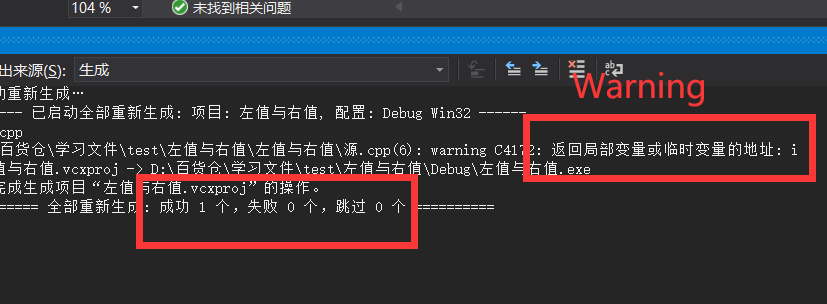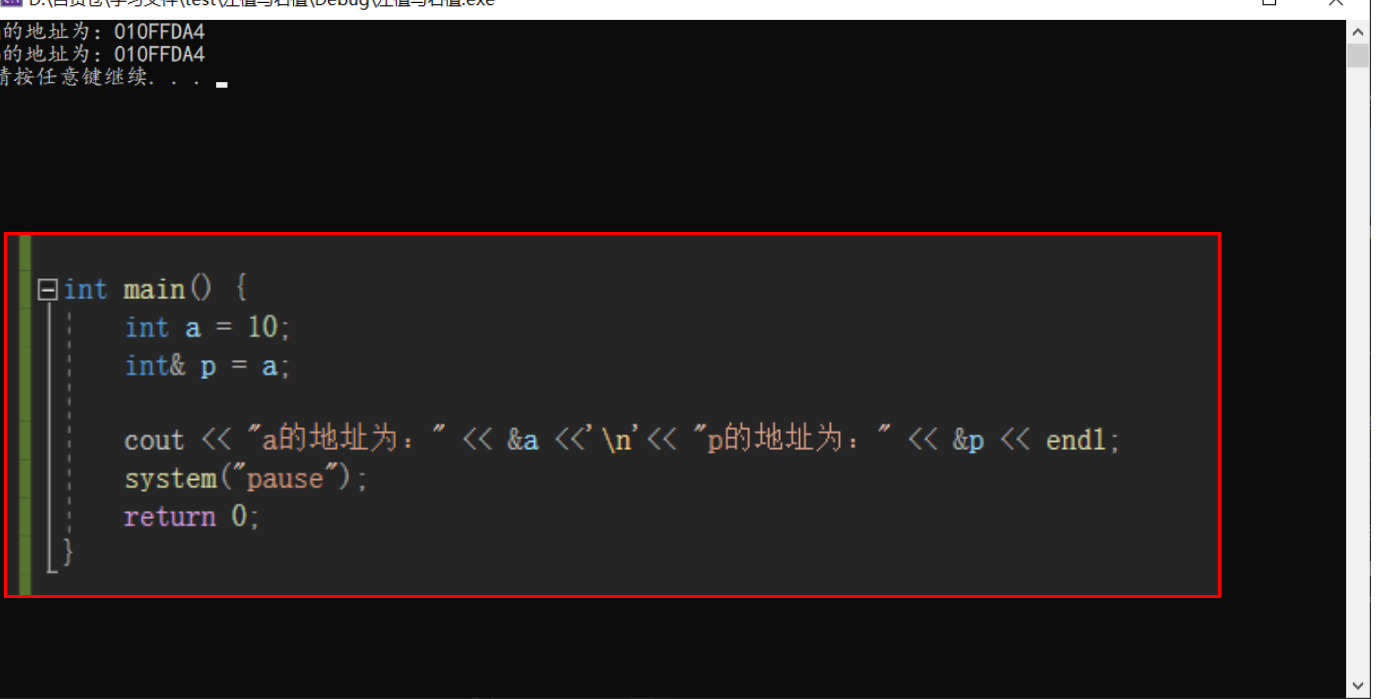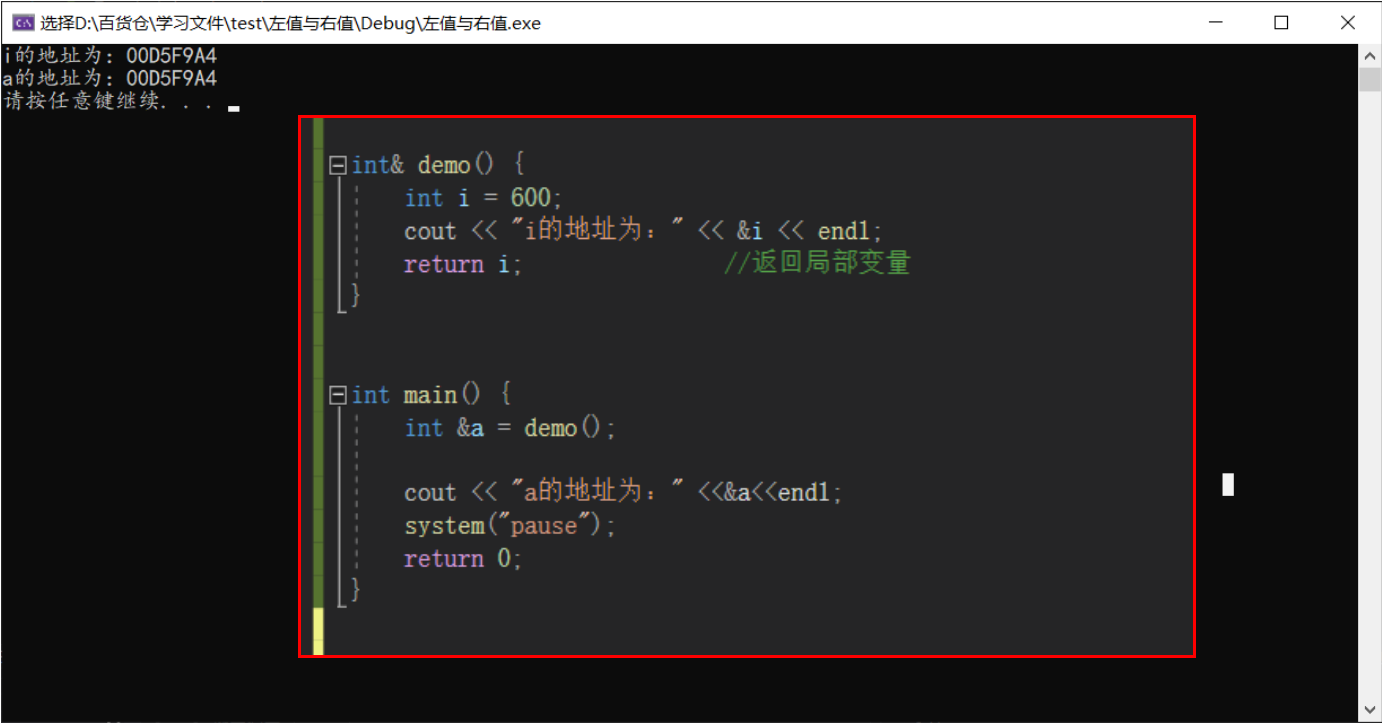我们现在更改a的值，然后再调用demo函数

int main() {
int &a = demo();
cout << "a的地址为：" <<&a<<endl;

a = 888;
demo();
cout << "再次调用demo(),a的值为：" << a << endl;
system("pause");
return 0;
}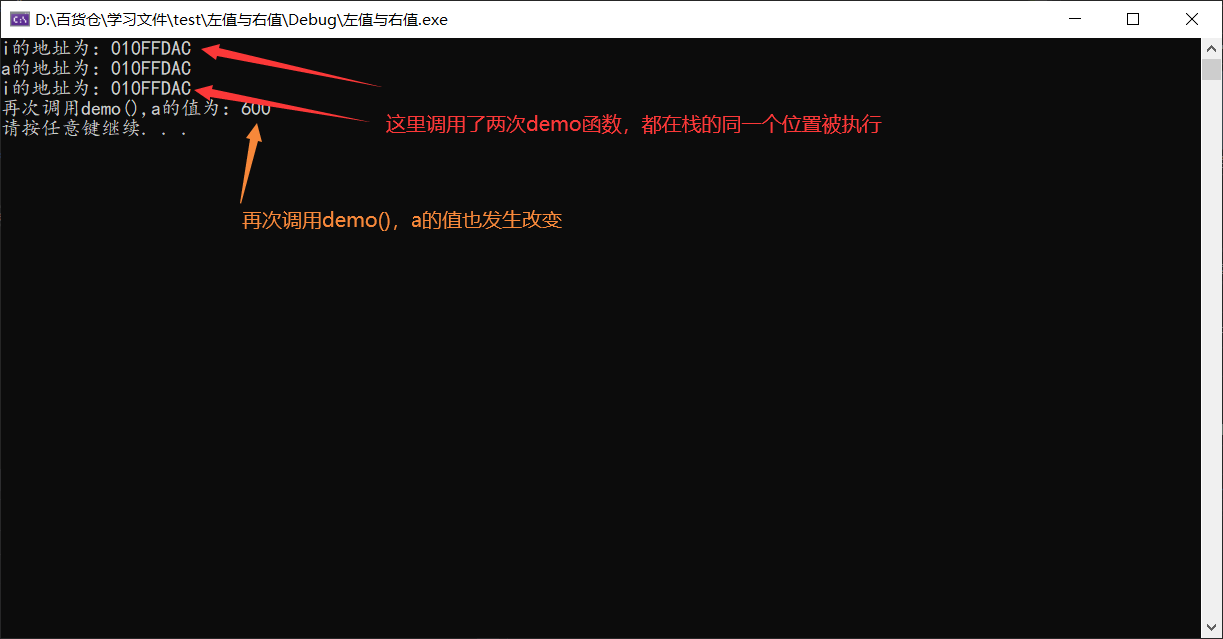#include<iostream>
#include<fstream>
using namespace std;

int demo1() {
int i = 100;
cout << "demo1()函数中，i的地址为：" << &i << endl;
return 0;
}

int& demo() {
int i = 600;
cout << "i的地址为：" << &i << endl;
return i;				//返回局部变量
}

int main() {
int &a = demo();
cout << "a的地址为：" <<&a<<endl;

a = 888;
demo1();
cout << "调用了demo1(),a的值为：" << a << endl;
system("pause");
return 0;
}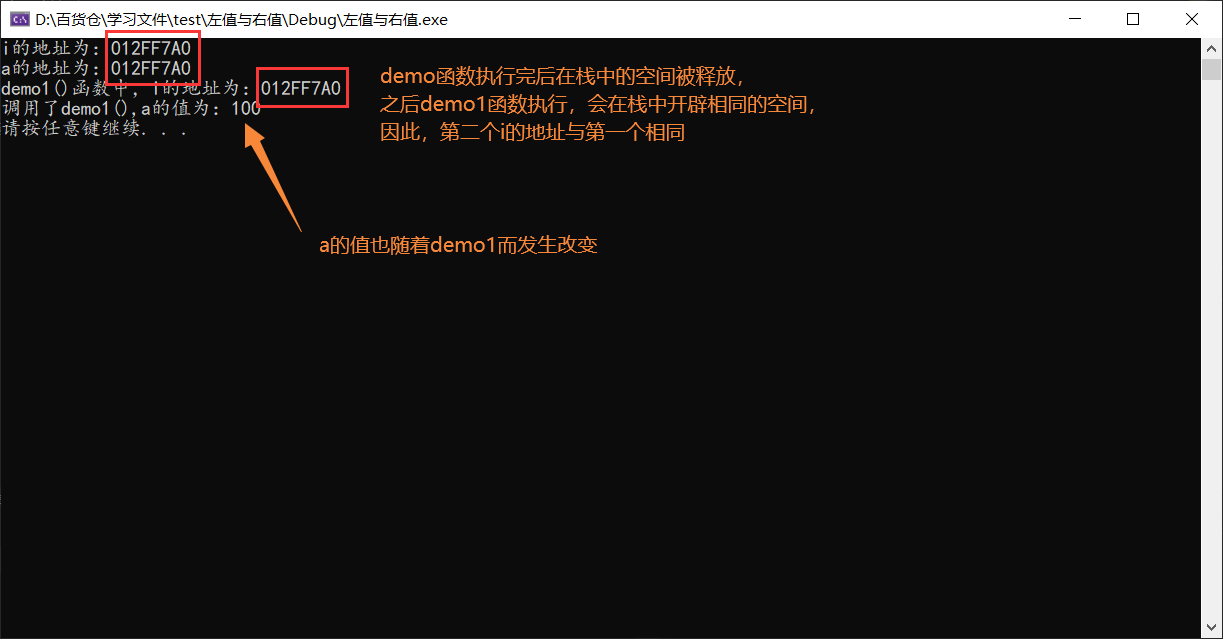2.返回局部变量作为左值

情况与上面类似

#include<iostream>
#include<fstream>
using namespace std;

int demo1() {
int i = 0;
cout << "demo1()函数中，i的地址为：" << &i << endl;
return 0;
}

int& demo(int **p) {
int i = 600;
*p = &i;
cout << "i的地址为：" << &i << " i的值为：" << i << endl;
return i;				//返回局部变量
}

int main() {
int* p = NULL;
demo(&p) = 888;
cout << "p的地址为：" << p << " p的值为：" << *p << endl;
demo1();
cout << "p的地址为：" << p << " p的值为：" << *p << endl;
system("pause");
return 0;
}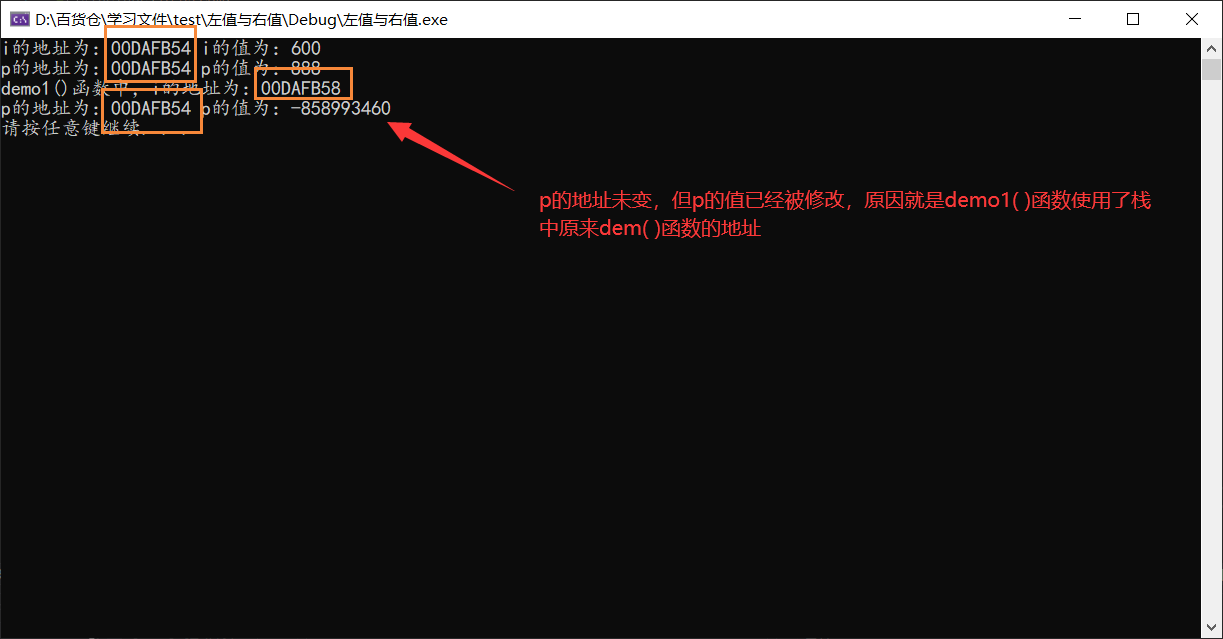总结：若返回栈变量（自动变量/临时变量），不能成为其它引用的初始值，不能作为左值使用，虽然编译器只会警告，但在实际开发中是非常危险的操作！

3.例如返回static或者全局变量就不出出现这种情况

#include<iostream>
#include<fstream>
using namespace std;

int& demo() {
static int i = 600;
cout << "i的地址为：" << &i << endl;
return i;				//返回静态变量
}

int main() {
int a = demo();
a = 888;
cout << "调用了demo(),a的值为：" << a << endl;
demo();
cout << "再次调用了demo(),a的值为：" << a << endl;
system("pause");
return 0;
}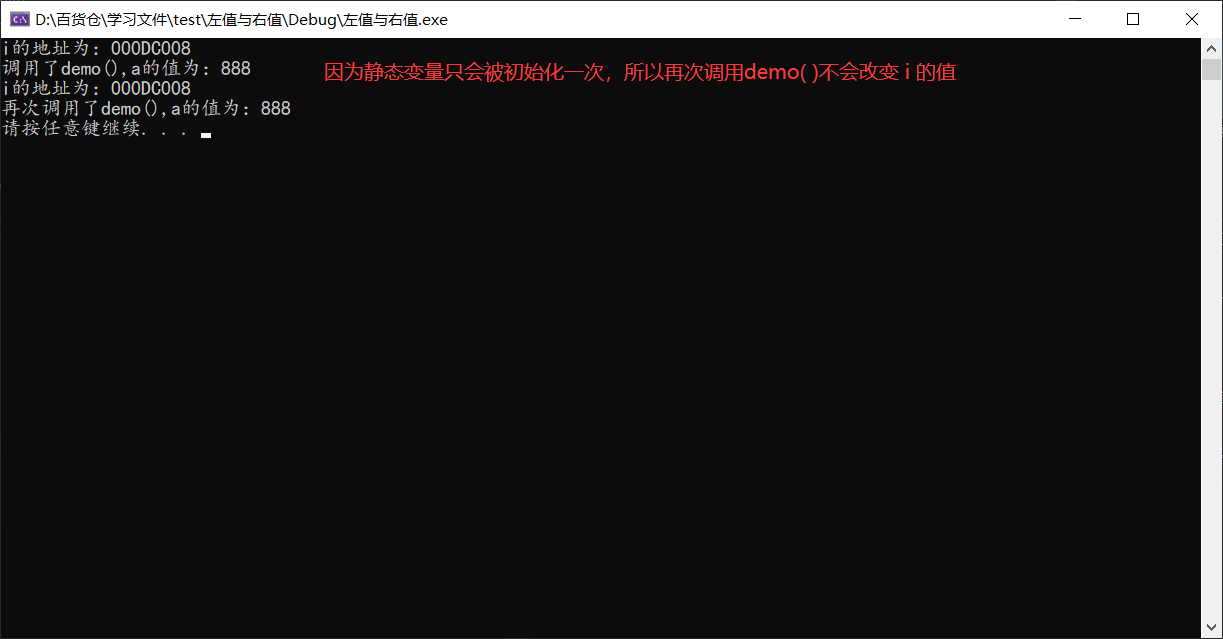4.C++11 _array容器用法

array容器概念

1. array 容器是 C++ 11 标准中新增的序列容器，简单地理解，它就是在 C++ 普通数组的基础上，添加了一些成员函数和全局函数。
1. array是将元素置于一个固定数组中加以管理的容器。
2. array可以随机存取元素,支持索引值直接存取， 用[]操作符或at()方法对元素进行操作，也可以使用迭代器访问
3. 不支持动态的新增删除操作
4. array可以完全替代C语言中的数组，使操作数组元素更加安全！
5. #include <array>

array特点

1. array 容器的大小是固定的，无法动态的扩展或收缩，这也就意味着，在使用该容器的过程无法增加或移除元素而改变其大小，它只允许访问或者替换存储的元素。
2. STL 还提供有可动态扩展或收缩存储空间的 vector 容器

array对象的构造

array采用模板类实现，array对象的默认构造形式

array<T，size> arrayT;    //T为存储的类型, 为数值型模板参数

//构造函数

#include<array>

array<int, 5> a1;     //一个存放5个int的array容器

array<float, 6> a2;   //一个存放6个float的array容器

array<student, 7> a3; //一个存放7个student的array容器

array的赋值

array 的赋值

a1.assign(0); //玩法一 改变array中所有元素(注：将被废弃，不推荐使用)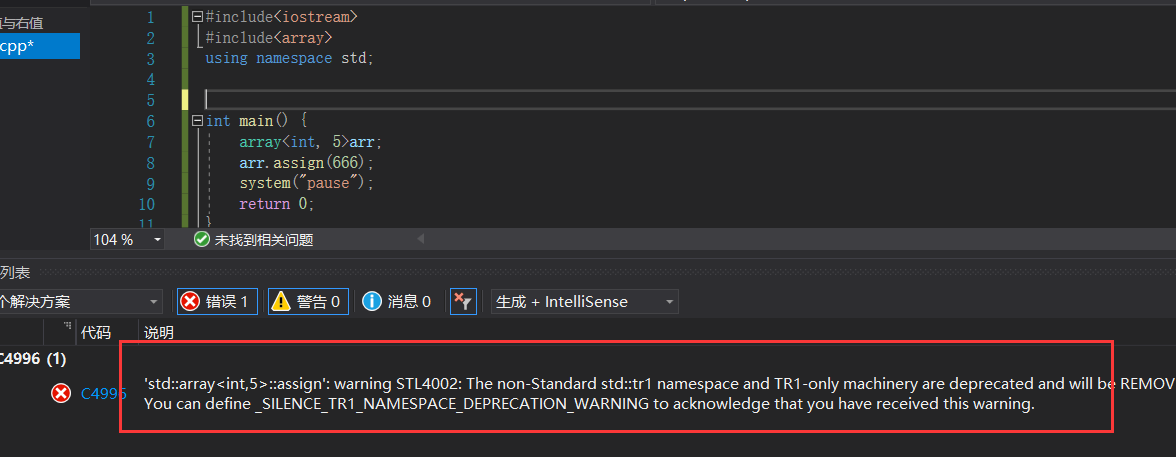#define _SILENCE_TR1_NAMESPACE_DEPRECATION_WARNING 1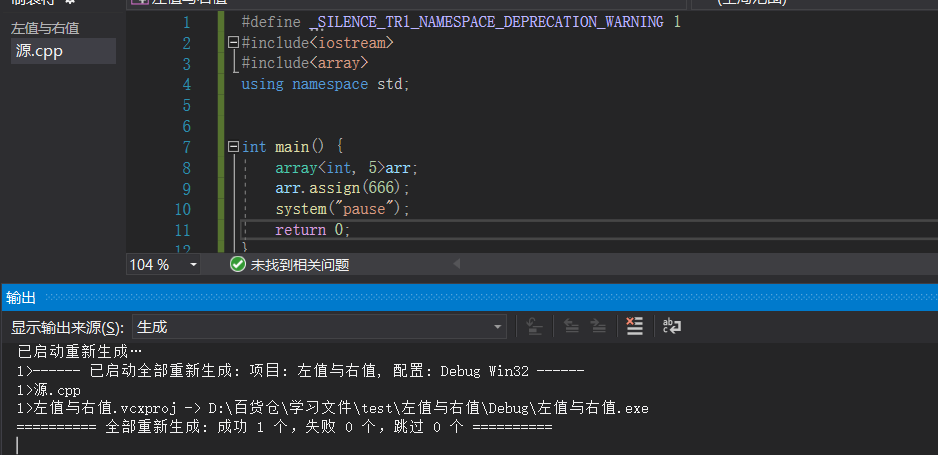a1.fill(666); //玩法二 用特定值填充array中所有元素

array<int, 4> test={1, 2, 3, 4};// 玩法三 定义时使用初始化列表，列表内的数量不能超过定义的大小

array<int, 4> test;

test={1,2,3,4};   //玩法四 定义后使用列表重新赋值

array<int, 4> a1,a2;

a1={1,2,3,4};

a2 = a1;//玩法五，赋值运算

a1.swap(a2);  //玩法六  和其它array进行交换

array的大小

array.size();    返回容器中元素的个数

array.empty();    判断容器是否为空,逗你玩的，因为容器是固定大小，永远为 false

array.max_size();   返回容器中最大元素的个数，数组是固定大小，这也是逗你玩的同size()。

array的数据存取

array 迭代器访问

#include<array>
using namespace std;
array.begin();         返回容器中第一个数据的迭代器。

array.end();          返回容器中最后一个数据之后的迭代器。

array.rbegin();        返回容器中倒数第一个元素的迭代器。

array.rend();         返回容器中倒数最后一个元素的后面的迭代器。

array.cbegin();        返回容器中第一个数据的常量迭代器。

array.cend();          返回容器中最后一个数据之后的常量迭代器。

array.crbegin();        返回容器中倒数第一个元素的常量迭代器。

array.crend();         返回容器中倒数最后一个元素的后面的常量迭代器。
#include<iostream>
#include<array>
using namespace std;

int main() {

array<int, 5> arrayInt = { 1,2,3,4,5 };

普通迭代器
for (array<int, 5>::iterator ite = arrayInt.begin(); ite != arrayInt.end(); ite++)
cout << *ite << "  ";

常量迭代器，常量迭代器无法修改
array<int, 5>::const_iterator ite = arrayInt.cbegin();

逆向迭代器，逆向迭代器也是++
for (array<int, 5>::reverse_iterator ite = arrayInt.rbegin(); ite != arrayInt.rend(); ite++)
cout << *ite << "  ";

}

set.rbegin()与set.rend()。略。

5.C++类型转换简介

旧式转型  C风格的强制类型

type  b  =  (  type  )  a

例如：

int i = 48;

char  c = (char ) i;

double  PI = 3.1415926;

int i = PI;   隐式转换

int i1 = (int) PI ; 强制类型转换

int * addr = (int*) 0x888888;

void *p;
int * int_arg = (int*) p;  强制转换

新式转型C++风格的类型转换提供了4种类型转换操作符来应对不同场合的应用。

type b = 类型操作符<type> ( a )

类型操作符= static_cast | reinterpreter_cast | dynamic_cast | const_cast

5.1.static_cas转换

静态类型转换（斯文的劝导，温柔的转换）（编译器会检查转换是否合理）。如int转换成char

char a = 'A';
int b = static_cast <int>(a);

• 用于类层次结构中基类（父类）和派生类（子类）之间指针或引用的转换。上行指针或引用(派生类到基类)转换安全，下行不安全（上行可以，下行不安全）
• 用于基本数据类型之间的转换，如把int转换成char，把int转换成enum。这种转换的安全性也要开发人员来保证。
• 把空指针转换成目标类型的空指针。
• 把任何类型的表达式转换成void类型

1.用于类层次结构中基类（父类）和派生类（子类）之间指针或引用的转换

class Animal {
public:
virtual void cry() = 0;
};

class dog :public Animal {
public:
void cry() {
cout << "小狗汪汪汪" << endl;
}
};

int main() {
dog* d = new dog();
Animal* animal = d;//子类对象赋给基类

使用static_cast指针转换
Animal* al = static_cast<Animal*>(d);

引用转换
dog d2;
Animal& a2 = static_cast<Animal&>(d2);
system("pause");
return 0;
}

class cat :public Animal {
public:
void cry() {
cout << "小猫喵喵喵" << endl;
}
};
int main() {
dog* d = new dog();
//上行转换安全
Animal* animal = static_cast<Animal*>(d);
//下行转换，这种也是安全的
d = static_cast<dog*>(animal);

//animal已经是dog类转过来的，再转成cat的就不安全的
cat* c;
c = static_cast<cat*>(animal);

system("pause");
return 0;
}

2.基本数据类型之间的转换

int a = 10;
char b = static_cast<char>(a);

3.把空指针转换成目标类型的空指针。

int* p = static_cast<int*>(NULL);

NULL在C++里定义的是void*04.把任何类型的表达式转换成void类型

int* p = new int;
void* vp = static_cast<void*>(p);

5.2.reinterpreter_cast 转换

重新解释类型(挂羊头，卖狗肉) 不同类型间的互转，数值与指针间的互转

type b = reinterpret_cast <type > ( a )

type 必须是一个指针、引用、算术类型、函数指针。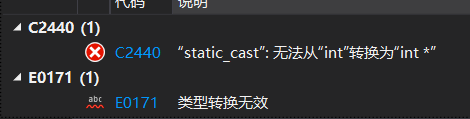int* p = reinterpret_cast<int*>(0x88888);
int val = reinterpret_cast<int>(p);

定义一个父类和两个派生类

class Animal {
public:
void cry() {
cout << "动物叫" << endl;
}
};

class cat :public Animal {
public:
void cry() {
cout << "小猫喵喵喵" << endl;
}
};

class dog :public Animal {
public:
void cry() {
cout << "小狗汪汪汪" << endl;
}
};

1.隐式上行  转换  与强制上行  转换

dog d1;
Animal* animal = &d1;
animal->cry();

//如果能用static_cast强转，static_cast优先
dog* d2 = reinterpret_cast<dog*>(animal);
dog* d3 = static_cast<dog*>(animal);

2.不同类型指针转换不能用static_cast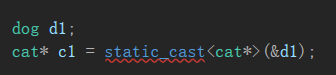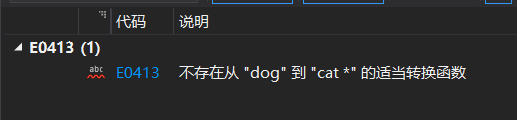3.但是可以使用reinterpre_cast转换

int main() {
dog d1;
cat* c1 = reinterpret_cast<cat*>(&d1);
c1->cry();

system("pause");
return 0;
}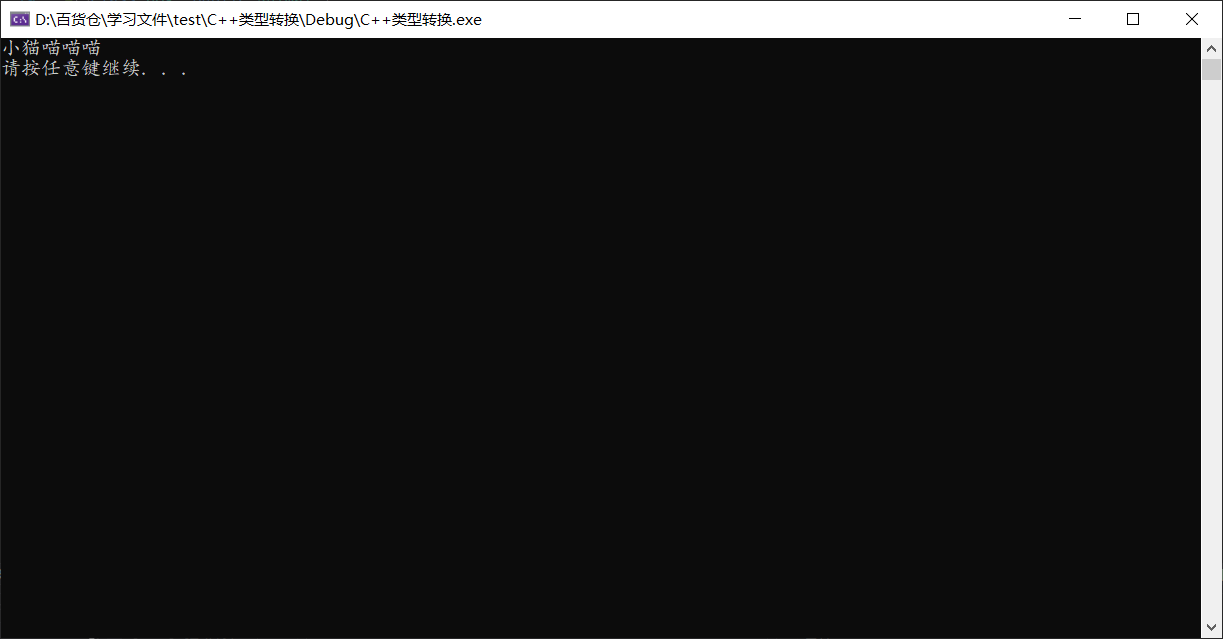4.引用的强制类型转换

dog d1;
Animal& animal = d1;
dog& d2 = reinterpret_cast<dog&>(animal);

reinterpreter_cast  再加一个 static_cast 就已经可以替换C语言的转换

5.3.dynamic_cast转换

动态类型转换

• 将一个基类对象指针cast到继承类指针，dynamic_cast 会根据基类指针是否真正指向继承类指针来做相应处理。失败返回null，成功返回正常cast后的对象指针；

#include<iostream>
using namespace std;

class Animal {
public:
//父类必须有虚函数
virtual void cry() = 0;
};

class cat :public Animal {
public:
void cry() {
cout << "小猫喵喵喵" << endl;
}
};

class dog :public Animal {
public:
void cry() {
cout << "小狗汪汪汪" << endl;
}
};

int main() {

dog d1;
Animal* animal = &d1;
cat* ca= dynamic_cast<cat*>(animal);
if (ca==NULL) {
cout << "这是只狗！" << endl;
}
else {
cout << "这是只猫" << endl;
}

system("pause");
return 0;
}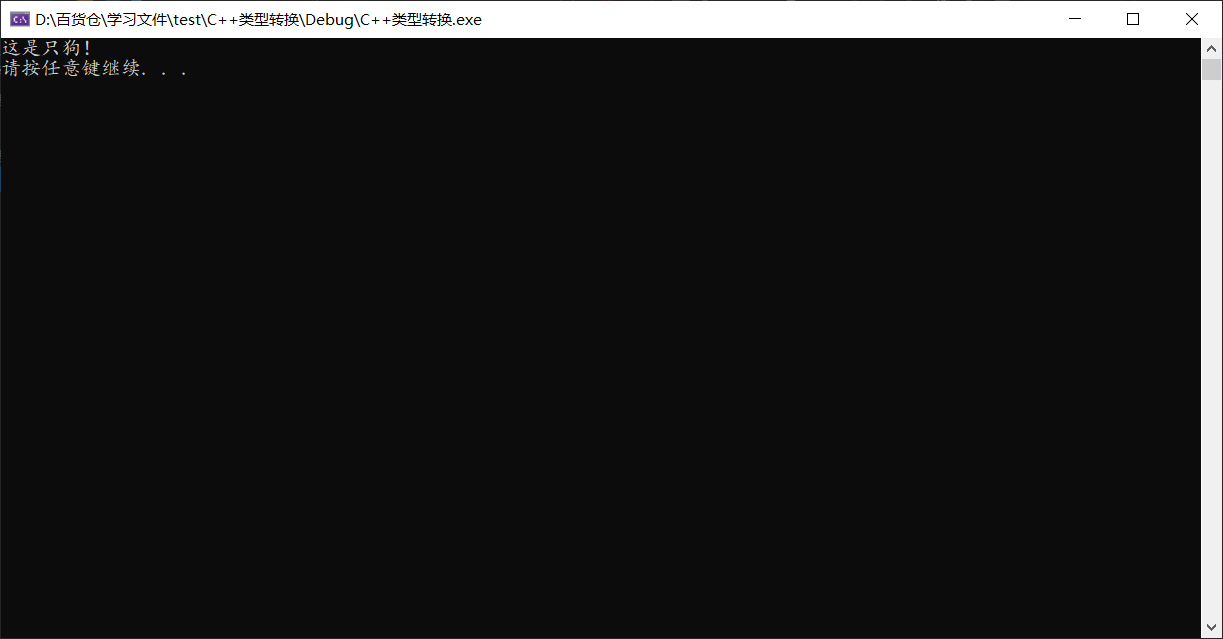int main() {
dog d1;
Animal& animal = d1;
dog& d2 = dynamic_cast<dog&>(animal);
system("pause");
return 0;
}

int main() {

dog d1;
Animal& animal = d1;
cat& d2 = dynamic_cast<cat&>(animal);
system("pause");
return 0;
}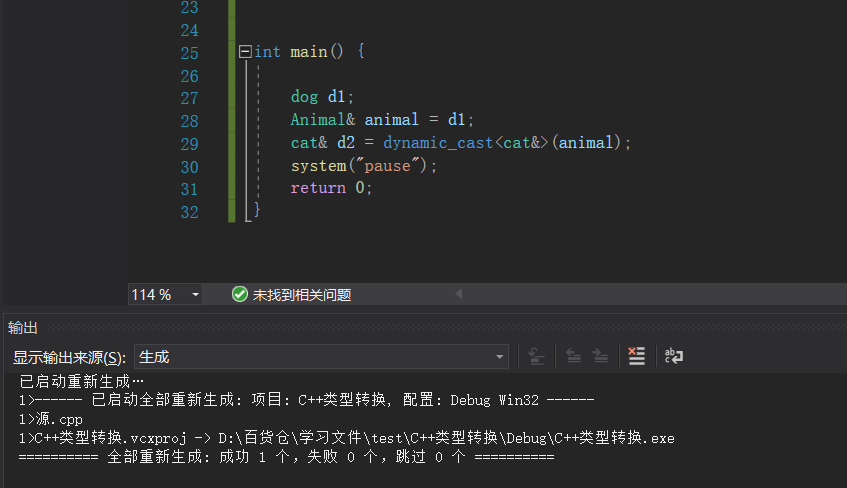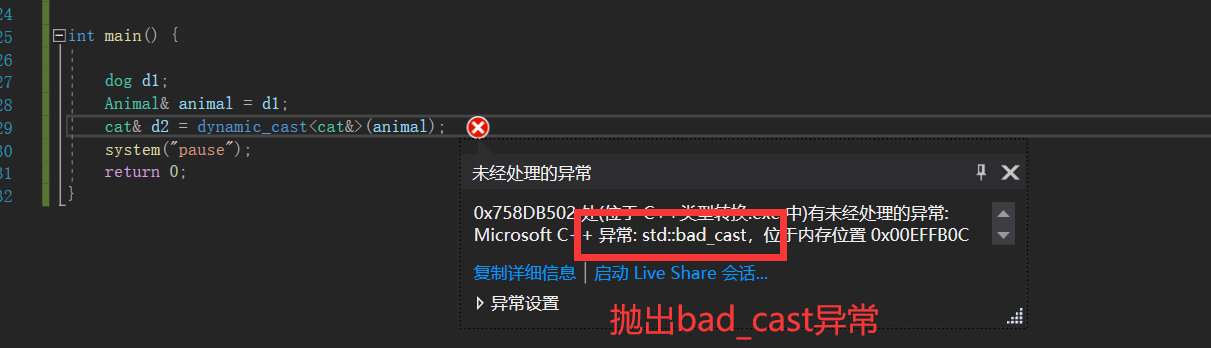int main() {

dog d1;
Animal& animal = d1;
try {
cat& d2 = dynamic_cast<cat&>(animal);
}
cout << "不是猫，应该是狗" << endl;
}

system("pause");
return 0;
}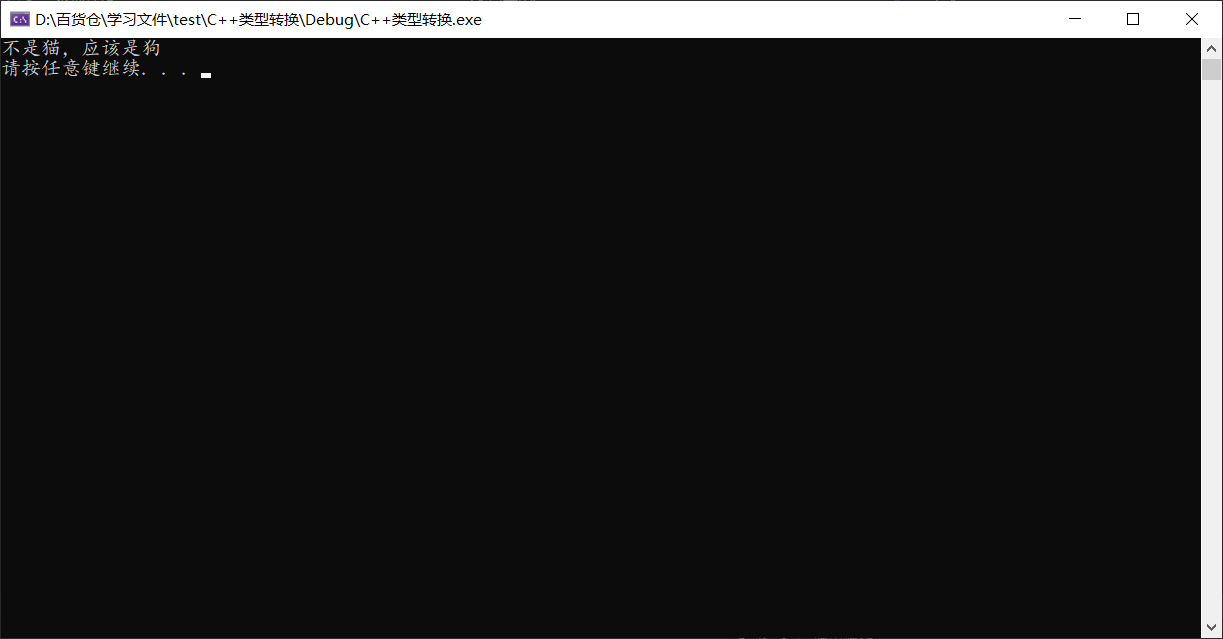5.4.const_cast 转换

去const属性。(仅针对于指针和引用)

例如

#include<iostream>
using namespace std;

void demo(const char* p) {
char* p1 = const_cast<char*>(p);
p1 = 'A';
}

int main() {
//字符数组
char p[] = "1234567";
demo(p);
cout << p << endl;
system("pause");
return 0;
}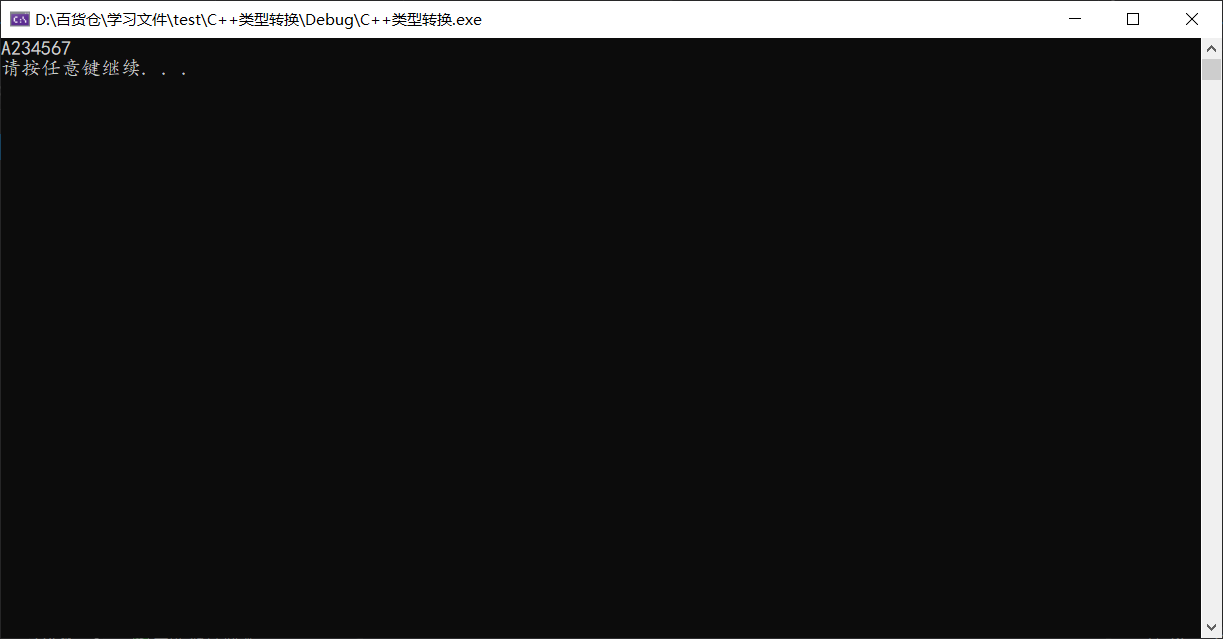int main() {
char p[] = "1234567";
const_cast<char*>(p) = 'A';
cout << p << endl;
system("pause");
return 0;
}

但是，对于常量字符串不能去掉const修改

#include<iostream>
using namespace std;

void demo(const char* p) {
char* p1 = const_cast<char*>(p);
p1 = 'A';
}

int main() {
const char *p = "1234567";
demo(p);
cout << p << endl;
system("pause");
return 0;
}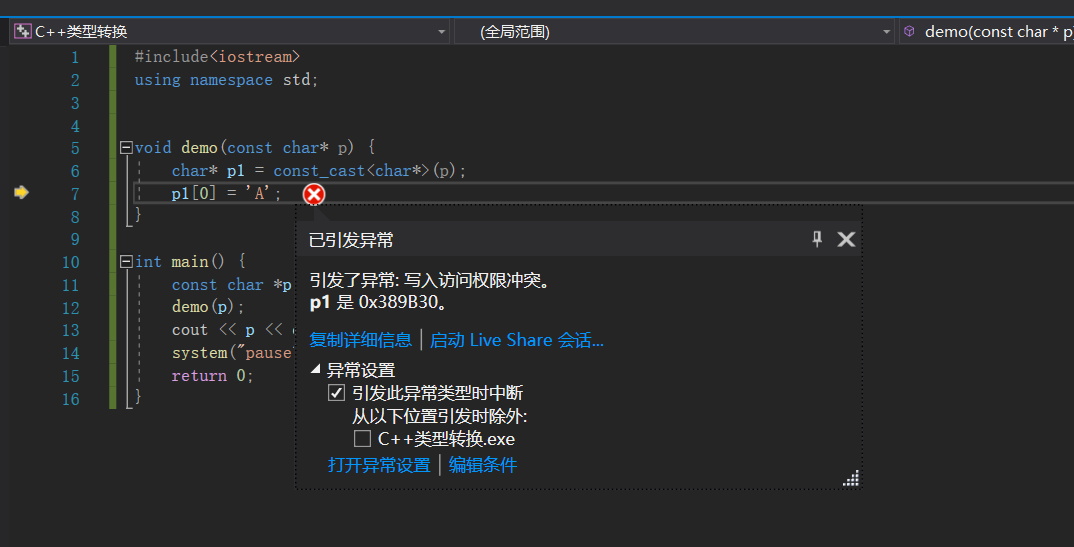5.2 类型转换使用建议

1）static_cast静态类型转换，编译的时c++编译器会做编译时的类型检查；隐式转换；

2）若不同类型之间，进行强制类型转换，用reinterpret_cast<>() 进行重新解释

建  议

C语言中  能隐式类型转换的，在c++中可用 static_cast<>()进行类型转换。因C++编译器在编译检查一般都能通过；C语言中不能隐式类型转换的，在c++中可以用 reinterpret_cast<>() 进行强制类型解释

总结：static_cast<>()和reinterpret_cast<>() 基本上把C语言中的 强制类型转换给覆盖，注意reinterpret_cast<>()很难保证移植性。

3）dynamic_cast<>()，动态类型转换，安全的虚基类和子类之间转换；运行时类型检查

4）const_cast<>()，去除变量的只读属性

C++大牛建议一般情况下，不建议进行类型转换；避免进行类型转换。

10-31565
03-09439606-053万+
02-2310万+
07-301639
09-281949
02-07793
08-272万+
11-168
11-30299
11-04313
04-133万+
02-0870
12-29463
01-20331
10-24447
07-2561
09-2789¥2 ¥4 ¥6 ¥10 ¥20余额支付 (余额：-- )扫码支付获取中扫码支付点击重新获取扫码支付1.余额是钱包充值的虚拟货币，按照1:1的比例进行支付金额的抵扣。
2.余额无法直接购买下载，可以购买VIP、C币套餐、付费专栏及课程。余额充值